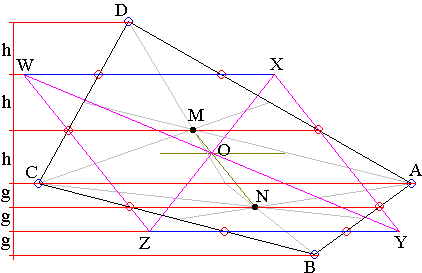# Wittenbauer's Parallelogram: What is it? A Mathematical Droodle

### This applet requires Sun's Java VM 2 which your browser may perceive as a popup. Which it is not. If you want to see the applet work, visit Sun's website at https://www.java.com/en/download/index.jsp, download and install Java VM and enjoy the applet.

 What if applet does not run?

Explanation### Wittenbauer's Parallelogram

Divide the sides of a quadrilateral ABCD into the thirds. Draw straight lines through the pairs of the division points next to each vertex. The four lines form a parallelogram bearing the name of F. Wittenbauer (1857-1922). The sides of the parallelogram are parallel to the diagonals of ABCD (which in fact explains why there's a parallelogram in the first place), exactly as the sides of Varignon's parallelogram.

Wittenbauer's theorem states that the center of Wittenbauer's parallelogram is exactly the barycenter - the center of gravity - of the quadrilateral ABCD. In other words, if ABCD is thought of as a physical thin piece of a uniformly distributed material, then the shape could be balanced at that point on a pointed stick or a needle.The drawing is suggestive in the case where ABCD is convex. In geometric terms the statement is as follows:

Let M, N, P, Q be the points of intersection of the medians of triangles ACD, ABC, BCD, and ABD, respectively. Then the point of intersection of MN and PQ coincides with the center - the point of intersection of the diagonals - of Wittenbauer's parallelogram.

The mechanical proof is the most natural. Consider triangles ACD and ABC. Their masses are proportional to their areas. Since they share the base AC, their masses are in the ratio of their altitudes to AC: 3h/3g = h/g. We may as well think of h and g as being the masses of those two triangles. In the case where ABCD is convex, its barycenter lies on the line joining the barycenters of the two triangles, the line MN.

The vertical distance, i.e., the distance in the direction perpendicular to AC, between M and N is h + g. The barycenter O' of ABCD is the point on MN which balances the mass h at M and the mass g at N. Obviously O' is at the (vertical) distance g from M and at the (vertical) distance h from N. If, as in the diagram h > g, the distance from O' to AC is h - g.

Now let's have a look at the Wittenbauer's parallelogram. The vertical distance between XW and YZ is 2(h + g). The distance from O to each of them is then h + g and to AC it's h - g. The points O and O' appear to lie on the same line parallel to AC. If we consider a second pair of triangles -- BCD and ABD -- a similar argument shows that the same points both lie on the line parallel to the second diagonal BD. Since two distinct lines intersect in at most 1 point, O and O' are bound to coincide.

When the quadrilateral is concave or even self-intersecting the theorem still holds, however the proof must obviously be modified.